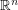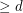# Kalai, Gil

## The Double Cap Conjecture ★★

Author(s): Kalai

Conjecture   The largest measure of a Lebesgue measurable subset of the unit sphere ofcontaining no pair of orthogonal vectors is attained by two open caps of geodesic radiusaround the north and south poles.

## Cube-Simplex conjecture ★★★

Author(s): Kalai

Conjecture   For every positive integer, there exists an integerso that every polytope of dimensionhas a-dimensional face which is either a simplex or is combinatorially isomorphic to a-dimensional cube.

Keywords: cube; facet; polytope; simplex